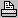Contents - Index

Deferred Revenue

Main Menu - Deal - Deferred Revenue

This is used to calculate deferred revenue using acquisition fees, RVI, & Gap values. It was specifically written for one ALMSys customer, but you may find that it could work in different situations.

Layout / How to
The whole idea of deferred revenue is to put off profits on a new lease and string them out over the life of the lease. This screen calculates deferred revenue in 3 steps. (Note: If you make any changes to the values on this screen, you must click the Calculate button to update the results.)

Step 1: Calculating the RVI Premium
RVI default information is stored on the Lease Company maintenance screen, RVI / Deferred Revenue page. If you use the Set Fields from Default Values button, this values will be pulled from the lease company defaults, as well as from this lease (or loan) itself.

The RVI Calculation is: Lease End Value  *  Insured Residual Percentage  *  Premium Percentage  *  (1 + Surplus Percentage)

Example: Lease end value is \$11,225.00, insured residual percentage is 75%, premium percentage is 0.6942%, and surplus percentage is 3%. The calulation would be:

11225 * 0.75 * 0.006942 * (1.03) = 60.2. So, \$60.20 is the RVI premium.

Step 2: Deferred Revenue Amount
The deferred revenue total amount calculation is: Acquisition Fee  -  Gap Fee  -  RVI Premium

Example: Acquition fee is \$490.00, gap fee is \$95.00, RVI premium is \$60.20. The calculation would be:

490 - 95 - 60.2 = 334.8. So, \$334.80 is the total deferred revenue amount.

Step 3: Monthly Revenue
The monthly revenue calculation is: Deferred Revenue Amount  /  Term

Example: Deferred revenue amount is \$334.80, term is 36 months. The calculation would be:

334.8 / 36 = 9.3. So, \$9.30 is the monthly deferred revenue amount. In order to make the total number of payments exactly equal to the total deferred revenue amount, ALMSys calculates the (monthly amt * (term - 1)),  subtracts that from the total deferred revenue, and places it into the Last Month Amt. field.

Amortization Schedule
Based on the Start Date entered, an amortization schedule is presented. Each deferred revenue payment is due by the end of each month. If a lease terminates early, the balance immediately becomes due.Reports
DealDefRev